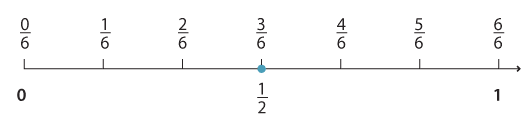## Content description

Compare fractions with related denominators and locate and represent them on a number line (ACMNA125)

Source: Australian Curriculum, Assessment and Reporting Authority (ACARA)

### Equivalent fractions

Equivalent fractions are located on the same place on the number line. The number line shows that $$\dfrac{3}{6}$$ and $$\dfrac{1}{2}$$ mark the same place. That is, $$\dfrac{3}{6}$$ and $$\dfrac{1}{2}$$ are equivalent fractions.Detailed decription

### Some important aspects of equivalence

• Equivalent fractions mark the same point on the number line.
• Equivalent fractions can be modelled using areas of rectangles.
• Equivalent fractions to a given fraction can be found by multiplying or dividing the numerator and the denominator of the fraction by the same whole number.
• In simple cases, fractions are 'related' if the denominator of one is a multiple of another. For example, the fractions $$\dfrac{1}{3} \text{and} \dfrac{5}{9}$$ have related denominators because 9 is a multiple of 3.
• It is possible for two fractions to be equivalent even when the denominators are not obviously related and the equivalence is not immediately obvious. For example, $$\dfrac{17}{34} \text{and} \dfrac{5}{10}$$ are both equivalent fractions since they are both equal to $$\dfrac{1}{2}$$.